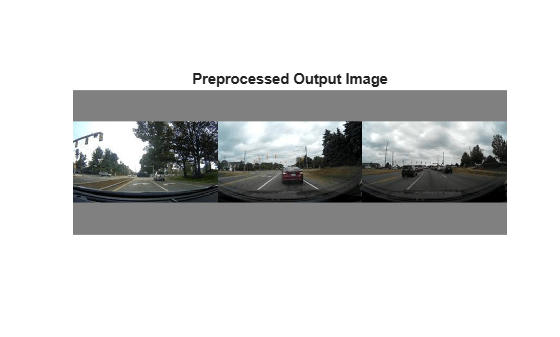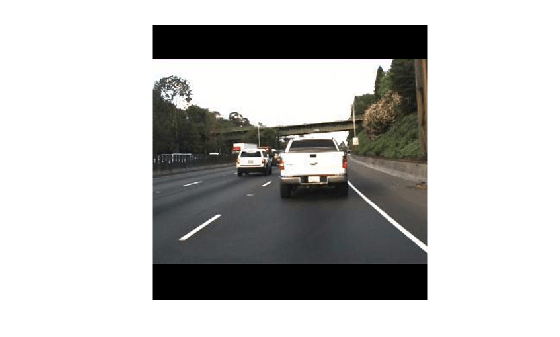preprocess

Preprocess training and test images

Description

example

outputData = preprocess(detector,trainingData) preprocesses the training data trainingData before using it to train the YOLO v3 object detector. The training images and the corresponding bounding boxes are stored in the trainingData. The preprocess function performs these operations:

• Rescales the intensity values of the training images to the range [0, 1].

• Resizes the training images to one of the nearest network input sizes and updates the bounding box coordinate values for accurate training. The function preserves the original aspect ratio of the training data.

example

outputImg = preprocess(detector,img) preprocesses the test images img for object detection using a YOLO v3 object detector. The preprocess function performs these operations:

• Rescales the intensity values of the test images to the range [0, 1].

• Resizes the test images to one of the nearest network input sizes and preserves the original aspect ratio of each test image.

[___,scaleInfo] = preprocess(___) returns information on the scale factor applied for image resizing, in addition to any combination of arguments from previous syntaxes.

Note

This function requires the Computer Vision Toolbox™ Model for YOLO v3 Object Detection. You can install the Computer Vision Toolbox Model for YOLO v3 Object Detection from Add-On Explorer. For more information about installing add-ons, see Get and Manage Add-Ons. To run this function, you will require the Deep Learning Toolbox™.

Examples

collapse all

Load a pretrained YOLO v3 object detector.

detector = yolov3ObjectDetector('tiny-yolov3-coco');

Load the training dataset into the workspace. The training data is a cell array that contains the images, bounding box values, and the class labels.

Resize the training images to the nearest network input size and rescale the intensity values by using the preprocess function.

outputData = preprocess(detector,trainingData);

Display the output image and the scale information used for resizing the image.

outputImg = outputData{1,1};
figure
imshow(outputImg)Display the input and the preprocessed values.

bboxIn = cell2table(trainingData,'VariableNames',{'Images','Bounding Boxes','Labels'})
bboxIn=3×3 table
Images               Bounding Boxes           Labels
_________________    ________________________    ___________

{224×399×3 uint8}    220    136     35     28    {'vehicle'}
{224×399×3 uint8}    175    126     61     45    {'vehicle'}
{224×399×3 uint8}    108    120     45     33    {'vehicle'}

bboxOut = cell2table(outputData,'VariableNames',{'Images','Bounding Boxes','Labels'})
bboxOut=3×3 table
Images               Bounding Boxes           Labels
__________________    ________________________    ___________

{416×416×3 single}    229    232     36     29    {'vehicle'}
{416×416×3 single}    182    222     64     46    {'vehicle'}
{416×416×3 single}    112    215     47     35    {'vehicle'}

Load a pretrained YOLO v3 object detector.

detector = yolov3ObjectDetector('tiny-yolov3-coco');

Resize the test image to the network input size and rescale the intensity values by using the preprocess function.

[outputImg,scaleInfo] = preprocess(detector,I);

Display the output image and the scale information used for resizing the image.

figure
imshow(outputImg)disp(scaleInfo)
PreprocessedImageSize: [416 416]
ScaleX: 0.7692
ScaleY: 0.5769

Input Arguments

collapse all

YOLO v3 object detector, specified as a yolov3ObjectDetector object.

Training data for YOLO v3 object detector, specified as a N-by-3 cell array that contains the images, bounding boxes, and the class labels. Each row is of the form [images bounding boxes labels]. N is the number of output layers in the network. The bounding boxes must be stored as a K-by-4 matrix of the form [x y width height]. K is the number of object classes.

Test images, specified as a numeric array of size M-by-N-by-C or M-by-N-by-C-by-T. M is the number of rows, N is the number of columns, and C is the number of color channels. The value of C is 1 for grayscale images and 3 for RGB color images. T is the number of test images in the array.

Data Types: single | double | int8 | int16 | int32 | int64 | uint8 | uint16 | uint32 | uint64

Output Arguments

collapse all

Preprocessed training data, returned as a N-by-3 cell array.

Data Types: cell

Preprocessed test images, returned as a numeric array of size P-by-Q-by-C or P-by-Q-by-C-by-T. P and Q are the number of rows and columns, respectively, in the preprocessed image. The datatype of the output preprocessed test images is same as that of the input test image.

Data Types: single | double | int8 | int16 | int32 | int64 | uint8 | uint16 | uint32 | uint64

Information about the scale factor for resizing the input images, returned as a structure with fields PreprocessedImageSize, ScaleX, and ScaleY.

• PreprocessedImageSize — Size of the output resized image.

• ScaleX — Scale factor for resizing an image in the X-direction (along the rows).

• ScaleY — Scale factor for resizing an image in the Y-direction (along the columns).

Data Types: struct